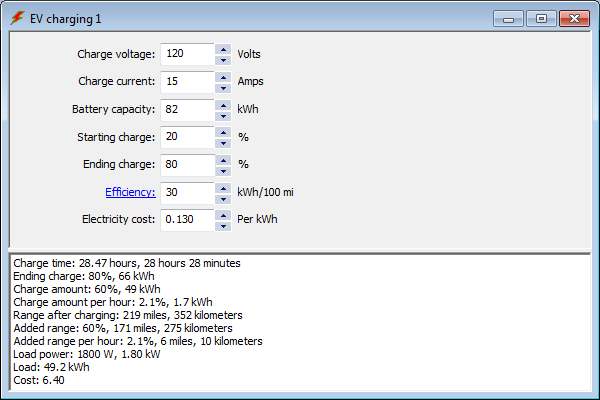## EV Charging Calculation 1

Use this calculation to determine EV charging time.Enter your charging voltage, charging current, battery capacity, starting charge, ending charge, efficiency, and electricity cost and calculate your charge time, total charge amount, charge amount per hour, range after charging, total added range, added range per hour, load power, load, and charging cost.

EV battery calculation

EV charging calculation 1

EV efficiency calculation

EV range calculation 1

EV range calculation 2

EV graph